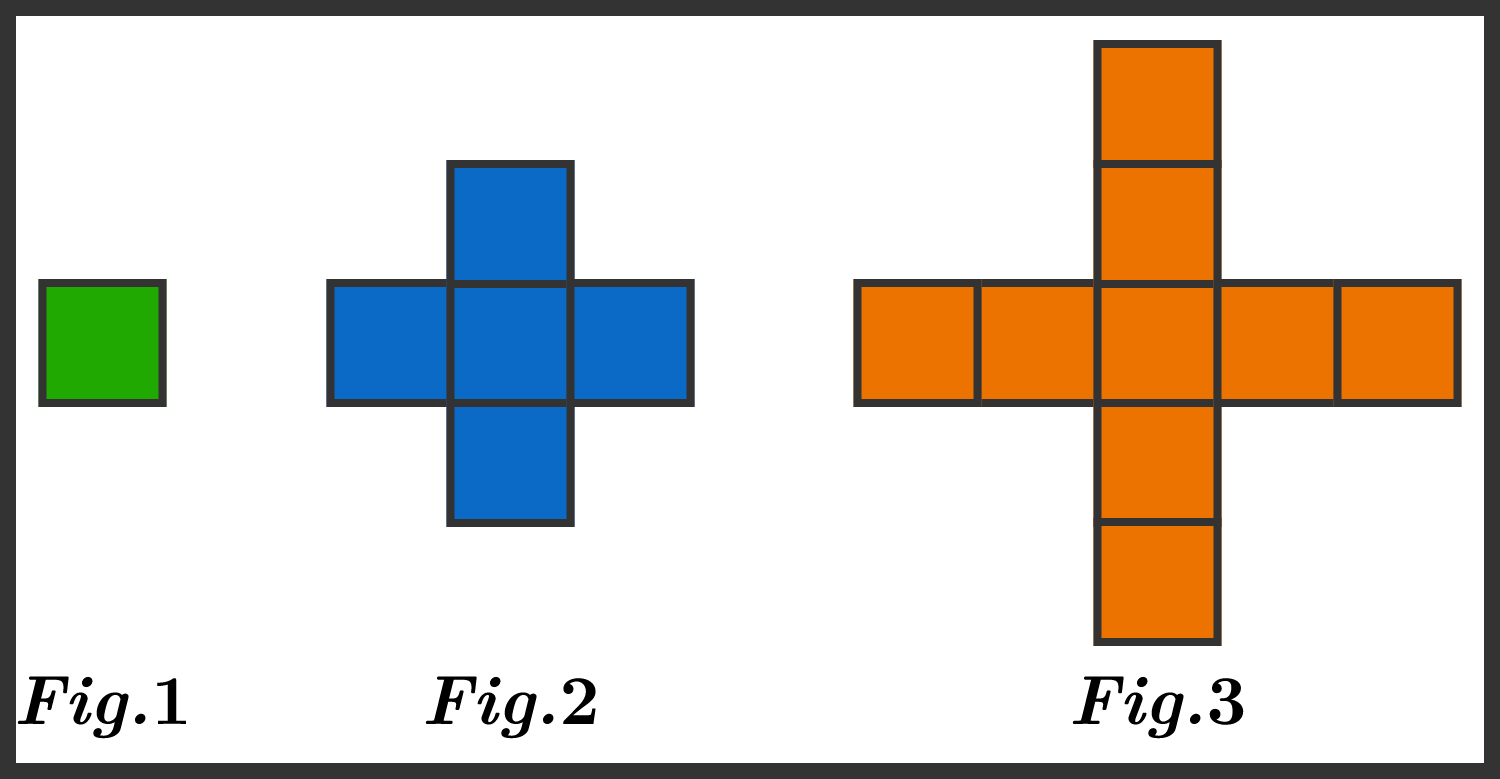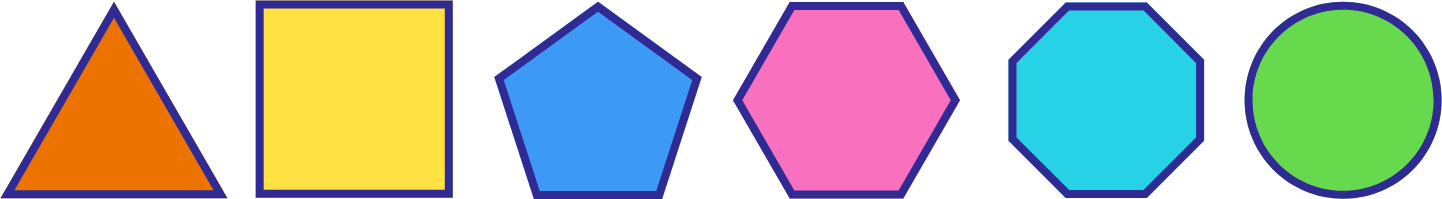Basic Mathematics

# Basic Mathematics Warmup: Level 1 Challenges

When I was two years old, my sister was half my age. If I am now sixty years old, how old is my sister?By tracing the edges, we can calculate that the perimeter of figure 1 is 4 units, and the perimeter of figure 2 is 12 units. What is the perimeter of figure 3?

Given that $a,$ $b,$ and $c$ are positive reals such that $a+b+c=6$, find the maximum possible value of $abc$.A triangle, a square, a pentagon, a hexagon, an octagon, and a circle all have the same perimeter.

Which one has the smallest area?

Note: All of the polygons are regular.

True or False?

If $x$ and $y$ are real numbers such that $\sqrt{x} = \sqrt{y}$, then

$x = y.$

×